@Team 2018-11-18T09:09:13.000000Z 字数 5106 阅读 1384

# 《Computer vision》笔记-ResNet（4）

石文华

1、前言：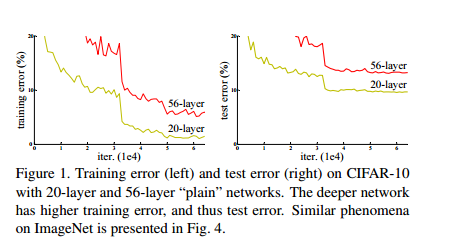2、核心结构：
resnet在2015年举办的ILSVRC中获得了分类任务第一名，同时，在 ImageNet detection, ImageNet localization,COCO detection, 和 COCO segmentation等任务中也是第一名。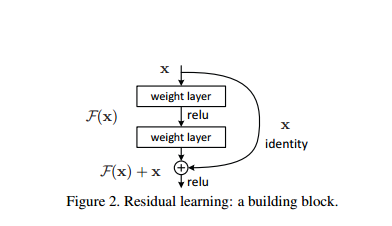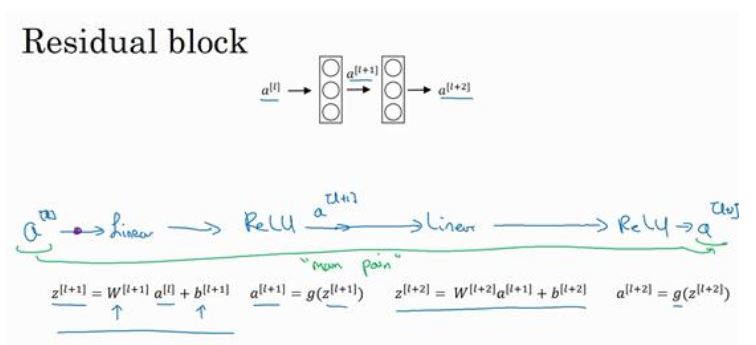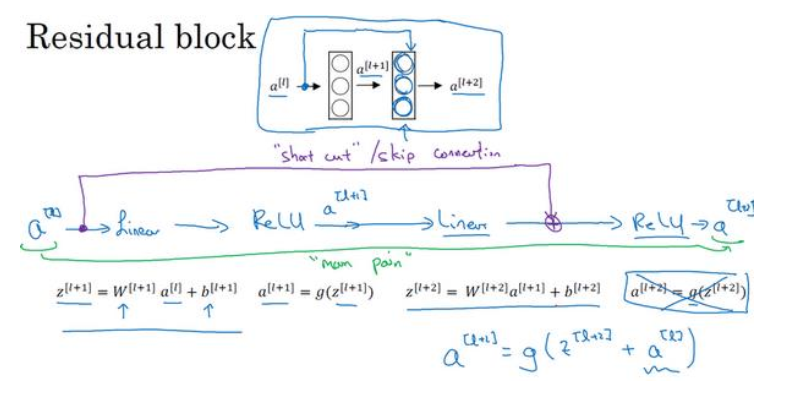Z[l+1]=W[l+1]*a[l]+b[l+1]
a[l+1]=g(Z[l+1])
Z[l+2]=W[l+2]*a[l+1]+b[l+2]
a[l+2]=g(Z[l+2])

3、网络结构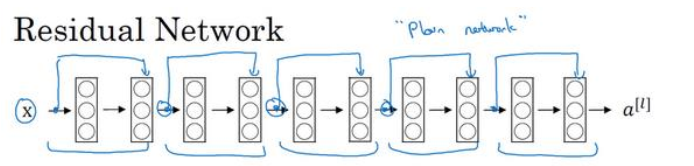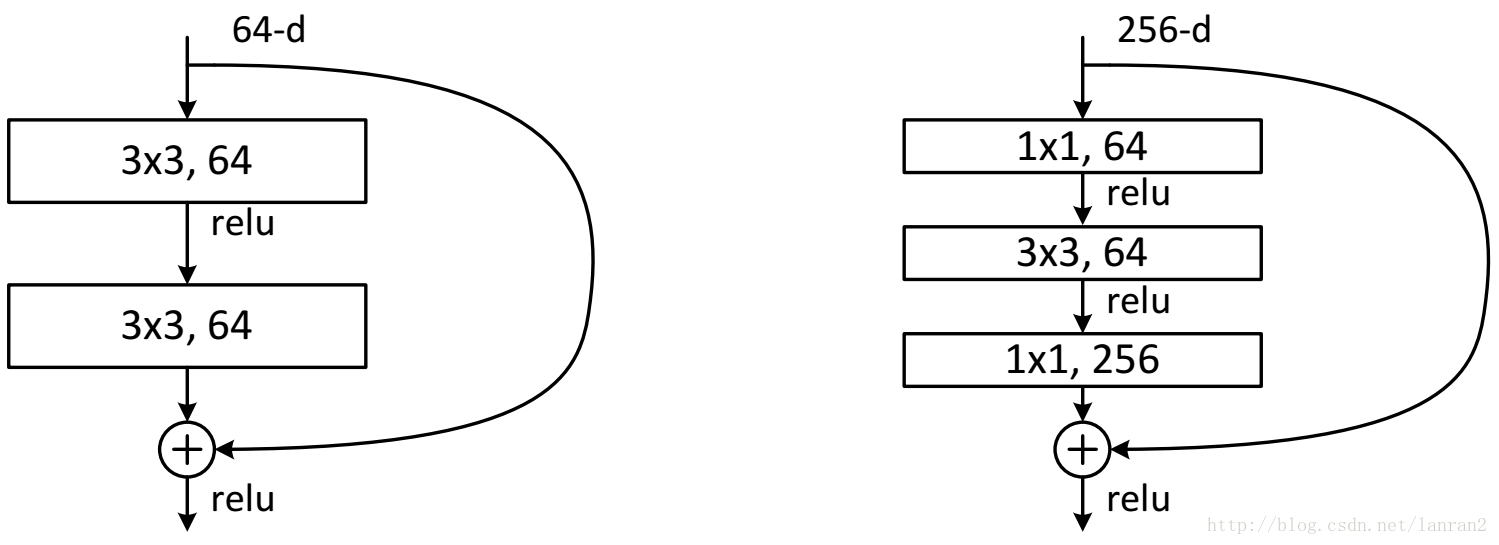4、改进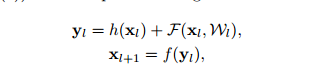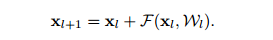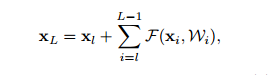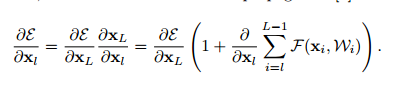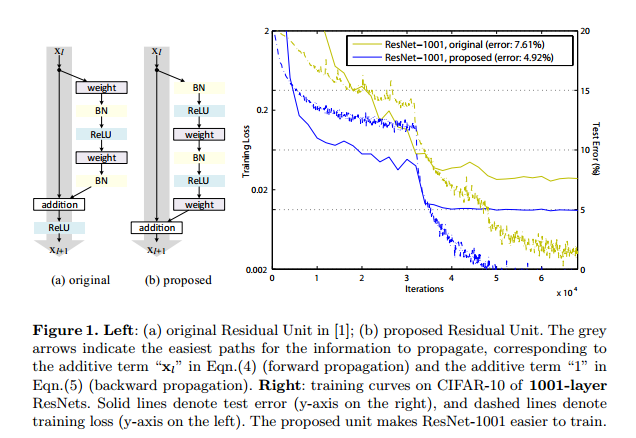5、代码：

import torch from torchvision import datasets,transformsimport osimport matplotlib.pyplot as pltimport time#transform = transforms.Compose是把一系列图片操作组合起来，比如减去像素均值等。#DataLoader读入的数据类型是PIL.Image#这里对图片不做任何处理，仅仅是把PIL.Image转换为torch.FloatTensor，从而可以被pytorch计算transform = transforms.Compose(    [        transforms.Scale([224,224]),        transforms.ToTensor(),        #transforms.Normalize(mean=[0.5,0.5,0.5],std=[0.5,0.5,0.5])    ])#训练集train_set = datasets.CIFAR10(root='drive/pytorch/Alexnet/', train=True, transform=transform, target_transform=None, download=True)#测试集test_set=datasets.CIFAR10(root='drive/pytorch/Alexnet/',train=False,download=True,transform=transform)trainloader=torch.utils.data.DataLoader(train_set,batch_size=32,shuffle=True,num_workers=0)testloader=torch.utils.data.DataLoader(test_set,batch_size=32,shuffle=True,num_workers=0)classes=('plane','car','bird','cat','deer','dog','frog','horse','ship','truck')(data,label)=train_setprint(classes[label])

X_example,y_example=next(iter(trainloader))print(X_example.shape)img=X_example.permute(0, 2, 3, 1)print(img.shape)import torchvisionimg=torchvision.utils.make_grid(X_example)img=img.numpy().transpose([1,2,0])import matplotlib.pyplot as pltplt.imshow(img)plt.show()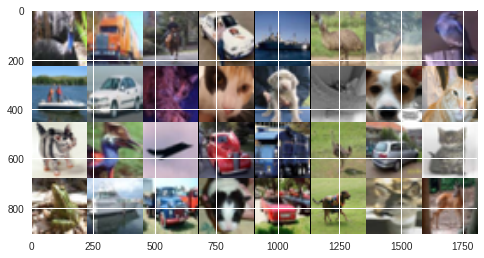from torchvision import modelsresnet=models.resnet50(pretrained=True)for parma in resnet.parameters():  parma.requires_grad = Falseresnet.fc = torch.nn.Linear(2048,10)cost=torch.nn.CrossEntropyLoss()optimizer=torch.optim.Adam(resnet.fc.parameters(),lr=0.0001)import torch.optim as optim          #导入torch.potim模块import timefrom torch.autograd import Variable   # 这一步还没有显式用到variable，但是现在写在这里也没问题，后面会用到import torch.nn as nnimport torch.nn.functional as Fepoch_n=5for epoch in range(epoch_n):  print("Epoch{}/{}".format(epoch,epoch_n-1))  print("-"*10)  running_loss = 0.0  #定义一个变量方便我们对loss进行输出  running_corrects=0  for i, data in enumerate(trainloader, 1): # 这里我们遇到了第一步中出现的trailoader，代码传入    inputs, labels = data   # data是从enumerate返回的data，包含数据和标签信息，分别赋值给inputs和labels    #inputs=inputs.permute(0, 2, 3, 1)    #print("hahah",len(labels))    y_pred = resnet(inputs)                # 把数据输进网络net，这个net()在第二步的代码最后一行我们已经定义了    _,pred=torch.max(y_pred.data,1)    optimizer.zero_grad()                # 要把梯度重新归零，因为反向传播过程中梯度会累加上一次循环的梯度    loss = cost(y_pred, labels)    # 计算损失值,criterion我们在第三步里面定义了    loss.backward()                      # loss进行反向传播，下文详解    optimizer.step()                     # 当执行反向传播之后，把优化器的参数进行更新，以便进行下一轮    # print statistics                   # 这几行代码不是必须的，为了打印出loss方便我们看而已，不影响训练过程    running_loss += loss.item()       # 从下面一行代码可以看出它是每循环0-1999共两千次才打印一次    running_corrects+=torch.sum(pred==labels.data)    if(i % 2 == 0):    # print every 2000 mini-batches   所以每个2000次之类先用running_loss进行累加      print("Batch{},Train Loss:{:.4f},Train ACC:{:.4f}".format(i,running_loss/i,100*running_corrects/(32*i)))• 私有
• 公开
• 删除